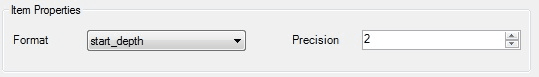﻿ Depth Interval

# Depth Interval

>SPM > SPM Desktop > Plans > Naming Schemas >

# Depth Interval

The Depth Interval Naming Schema allows the incorporation of a sample's depth into a sample's code. To learn how to create samples at different depths, read SPM - Setting Sample Depth for Planned Samples.

Sample depth can be represented in the following ways:

START_DEPTH: actual numerical value of start depth

END_DEPTH: actual numerical value of end depth

depthAsDigit: sequence number of a sample in the sampling interval, represented by a number, starting at 1

depthAsLetter: sequence number of a sample in the sampling interval, represented by a letter, starting at A

The example below demonstrates values for depth interval for each available setting. There are three samples for location MW1, taken every two feet, starting at zero.

Resulting Value for Depth Interval

Sample Parameters

START_DEPTH

END_DEPTH

depthAsDigit

depthAsLetter

Sample #1, start_depth = 0, end_depth = 2

0

2

1

A

Sample #2, start_depth = 2, end_depth = 4

2

4

2

B

Sample #3, start_depth = 4, end_depth = 6

4

6

3

C

The type of depth interval can be selected from the item's properties Format drop-down.Precision numerical box allows to define:

Format

Precision use

START_DEPTH

number of digits after decimal point, trailing zeros added if necessary

END_DEPTH

number of digits after decimal point, trailing zeros added if necessary

depthAsDigit

total number of digits, leading zeros added if necessary